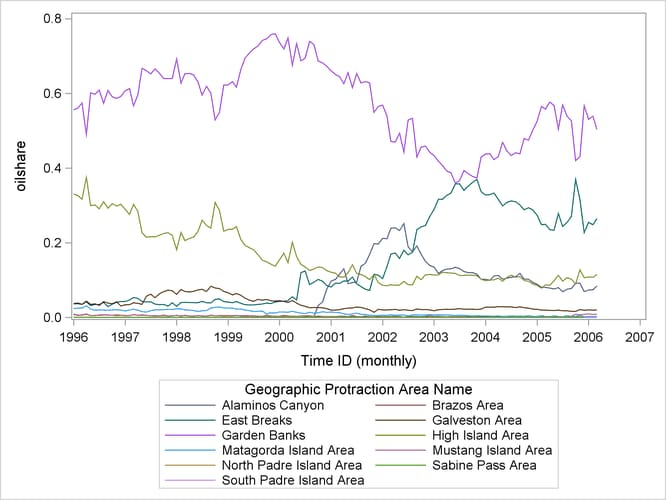# The TIMEDATA Procedure

### Example 30.3 Using Auxiliary Data Sets with PROC TIMEDATA

This example demonstrates the use of the AUXDATA= option in PROC TIMEDATA. The data set `Sashelp.Gulfoil` contains oil and gas production data from the Gulf of Mexico. The variables `RegionName` and `ProtractionName` can be used to define a time series hierarchy of interest. Suppose you want to generate two new series that contain the protraction’s share of oil and gas production for its associated region at each time index.

You first use PROC TIMESERIES to perform temporal aggregation (accumulation) of the time series for the `RegionName` level.

```proc timeseries data=sashelp.gulfoil
out=byregion(rename=(oil=roil gas=rgas));
by regionname;
id date interval=month accumulate=total notsorted;
var oil gas;
run;
```

You can then use PROC TIMEDATA with the AUXDATA= option to compute the share of oil and gas production contributed by each protraction within its associated region. PROC TIMEDATA reads a monthly time series for each (`RegionName`, `ProtractionName`) group for the variables `Oil` and `Gas` from `Sashelp.Gulfoil`. Two new series are produced in the variables `Oilshare` and `Gasshare` that respectively contain the protraction’s share of the oil and gas production at the region level of the hierarchy (given by variables `Roil` and `Rgas`). Those share variables are specified in the OUTARRAY statement for inclusion in the OUTARRAY= data set (`Work.Shares`). This example relies on the capability of the AUXDATA= feature to perform partial BY group matching. The time series that are acquired for the variables `Roil` and `Rgas` are the result of matching on the `RegionName` BY variable from the data set `Work.Byregion` with the `RegionName` variable from the BY groups that are acquired from the `Sashelp.Gulfoil` data set.

```proc timedata data=sashelp.gulfoil
auxdata=byregion
out=_null_
outarray=shares;
by regionname protractionname;
outarray oilshare gasshare;
var oil gas roil rgas;
id date interval=month accumulate=total;
do i=1 to _length_;
oilshare[i] = oil[i] / roil[i];
gasshare[i] = gas[i] / rgas[i];
end;
run;
```

The following code demonstrates that the computed shares sum to 1 for each time index in the resulting `Oilshare` and `Gasshare` series. PROC TIMESERIES is used to accumulate the shares for these respective variables from the data set `Work.Shares` and the accumulated share series at the `RegionName` level are stored to the data set `Work.Rshares` with variable names `Oilsum` and `Gassum`, respectively. The summary from PROC MEANS for the distinct values of `RegionName` shows that per-time totals for both share series sums to 1.

```proc timeseries data=shares
out=rshares(rename=(oilshare=oilsum gasshare=gassum));
by regionname;
id date interval=month accumulate=total notsorted;
var oilshare gasshare;
run;
proc means data=rshares;
by regionname;
var oilsum gassum;
run;
```

Output 30.3.1: Validation of Oil and Gas Shares by Region

The MEANS Procedure

Variable N Mean Std Dev Minimum Maximum
oilsum
gassum
 123 123
 1 1
 0 0
 1 1
 1 1

Variable N Mean Std Dev Minimum Maximum
oilsum
gassum
 123 123
 1 1
 0 0
 1 1
 1 1

You might also want to plot the share series. The following code produces a graph that overlays the protraction level share series for oil production for the Western region.

```proc sgplot data=shares(where=(RegionName='Western'));
series x=Date y=OilShare/group=ProtractionName;
run;
```

Output 30.3.2: Protraction Share of Oil Production for Western Region